# Transformer-XL及XLNet论文笔记

### Transformer-XL

#### 简介

• 引入循环机制（Reccurrence，让上一segment的隐含状态可以传递到下一个segment）。
• 提出一种新的相对位置编码方法，避免绝对位置编码在循环机制下的时序错乱。

#### 模型框架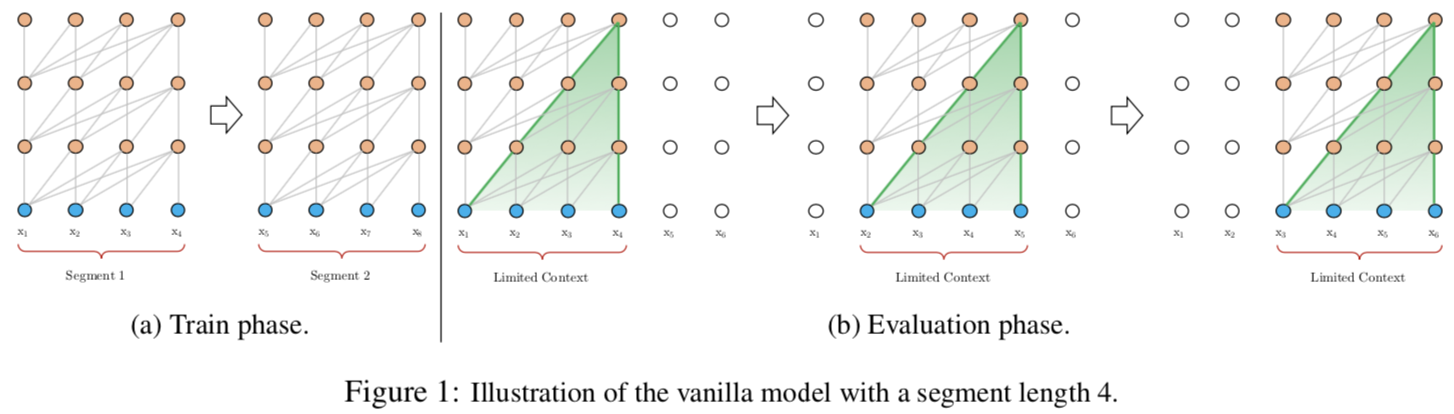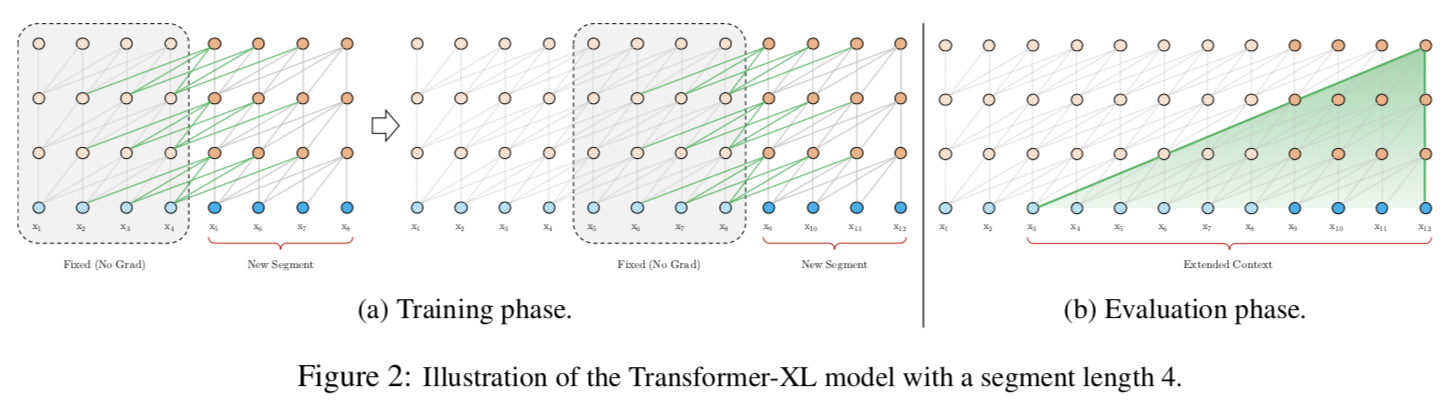##### Segment-Level Recurrence with State Reuse$\widetilde{h}_{\tau + 1}^{n-1}=[SG(h_{\tau}^{n-1})\circ h_{\tau + 1}^{n-1}], \\ q_{\tau + 1}^{n},k_{\tau + 1}^{n},v_{\tau + 1}^{n}=h_{\tau + 1}^{n-1}W_{q}^{T},\widetilde{h}_{\tau + 1}^{n-1}W_{k}^{T},\widetilde{h}_{\tau + 1}^{n-1}W_{v}^T, \\ h_{\tau + 1}^{n}=TransformerLayer(q_{\tau + 1}^{n},k_{\tau + 1}^{n},v_{\tau + 1}^{n})$$SG(\cdot)$表示梯度不回传（stop-gradient，fixed and cached），$[h_u\circ h_v]$表示向量在length维度拼接，这里其实就是将上一segment的内容考虑进来。另外，值得注意的一点是，这里和RNN的循环是在水平维度扩展不同（same-layer），这里是在垂直维度进行树的构建（between-layers），所以最大依赖长度和层深是线性关系，比如$O(N\times L)$，可以对照图2-b。论文说这和以前训练RNN-LMs的truncated BPTT长的比较像，其实我觉得更像去年提出的TCN（时间卷积网络），我很怀疑是不是从那里得到的启发，网络图太像了，后边找机会介绍一下TCN，在语音合成方面有很好的应用。多说一下，这里说的相像，都是网络图上的相似，但实际上是很不同的，包括这里的梯度不回传，和马上说到的相对位置编码。

##### Relative Positional Encodings$A_{i,j}^{abs}=\underbrace{E_{x_i}^TW_{q}^TW_kE_{x_j}}_{(a)} + \underbrace{E_{x_i}^TW_q^TW_kU_j}_{(b)}+\underbrace{U_i^TW_q^TW_kE_{x_j}}_{(c)}+\underbrace{U_i^TW_q^TW_kU_j}_{(d)}$$A_{i,j}^{ref}=\underbrace{E_{x_i}^TW_q^TW_{k,E}E_{x_j}}_{(a)}+\underbrace{E_{x_i}^TW_q^TW_{k,R}R_{i-j}}_{(b)}+\underbrace{u^TW_{k,E}E_{xj}}_{(c)}+\underbrace{v^TW_{k,R}R_{i-j}}_{(d)}$

•$(b)(d)$两项中，将所有绝对向量$U_j$转为相对位置向量$R_{i-j}$，和vanilla Transformer一样，这是个固定的编码向量，不需要学习。
•$(c)$项，将查询的$U_i^TW_q^T$向量转为一个需要学习的参数向量$u$，因为在考虑相对位置的时候，不需要查询的绝对位置$i$，因此对于任意的$i$，都可以采用同样的向量。同理，在$(d)$这一项中，也将查询的$U_i^TW_q^T$向量转为另一个需要学习的参数向量$v$，区分对待主要是和第3点结合。
• 将键的权重变换矩阵$W_k$分为$W_{k,E}$$W_{k,R}$两个矩阵，分别得到content-based的键向量、location-based的键向量，更加细致。

•$(a)$表示基于内容的寻址，即没有考虑位置编码的原始分数
•$(b)$表示内容相关的位置偏差，即相对于当前内容的位置偏差
•$(c)$表示全局的内容偏置，从内容层面衡量键的重要性
•$(d)$表示全局的位置偏置，从相对位置层面衡量键的重要性

2018年Shaw et al提出过类似的相对位置编码，相比这里的方案，主要少了$(c)$$(d)$两项，另外也不是采用Transformer最初提出来时的固定编码（正余弦）方案，而是使用一个可训练学习的矩阵$R$代替$W_kR$。后面实验时，会比较这两种方法。

#### 实验分析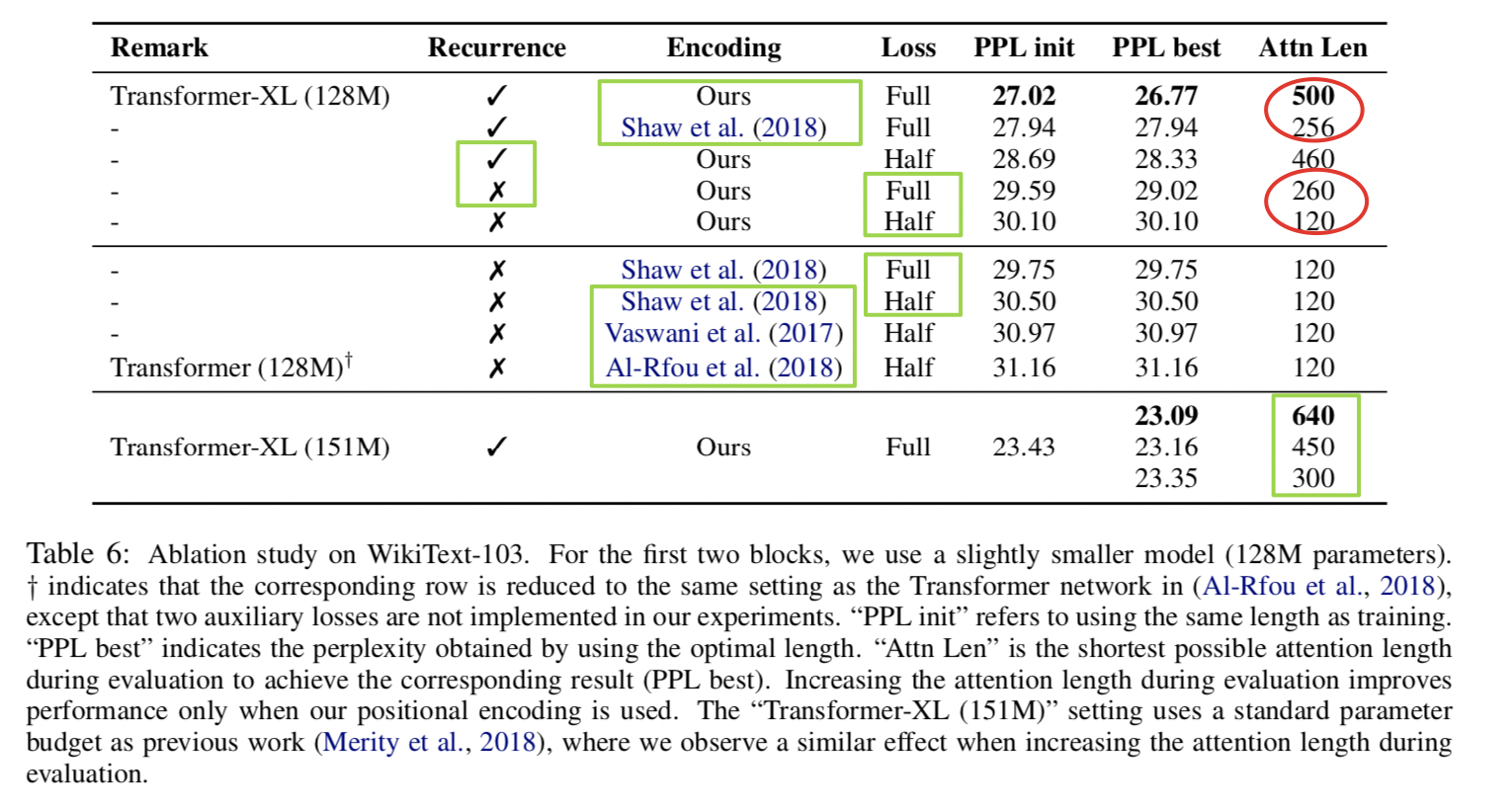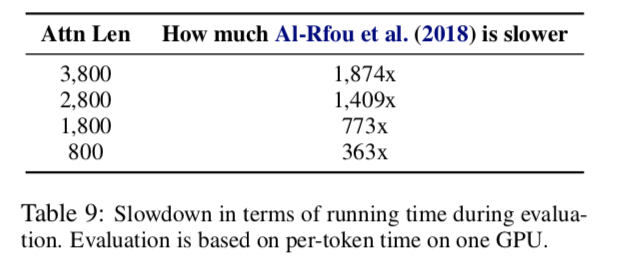#### 附录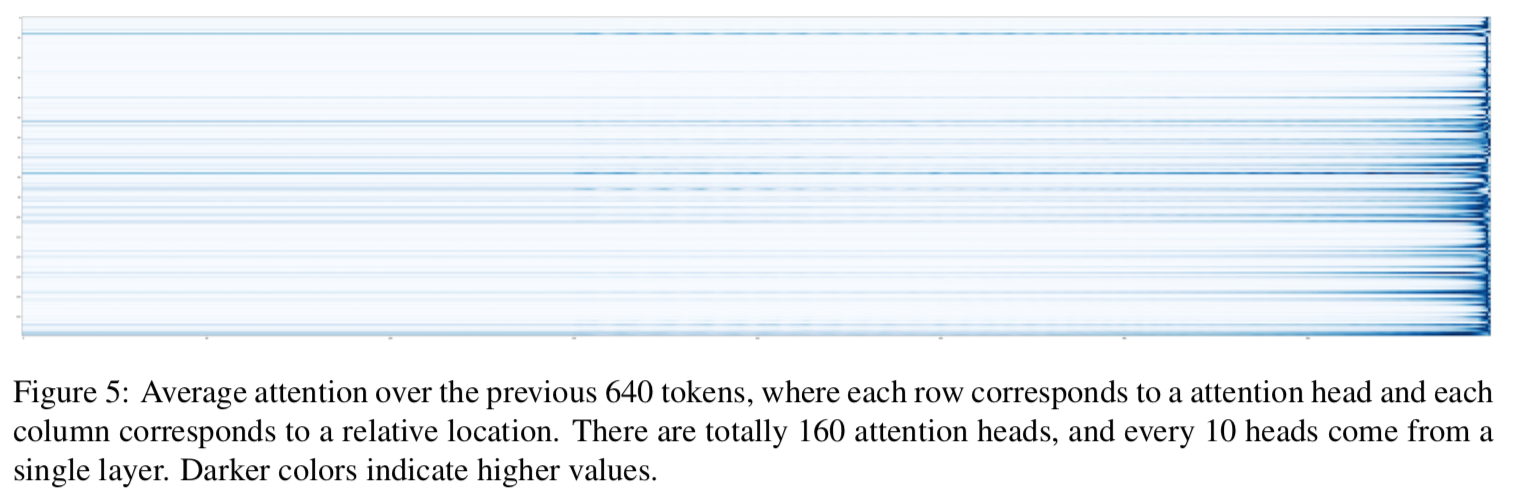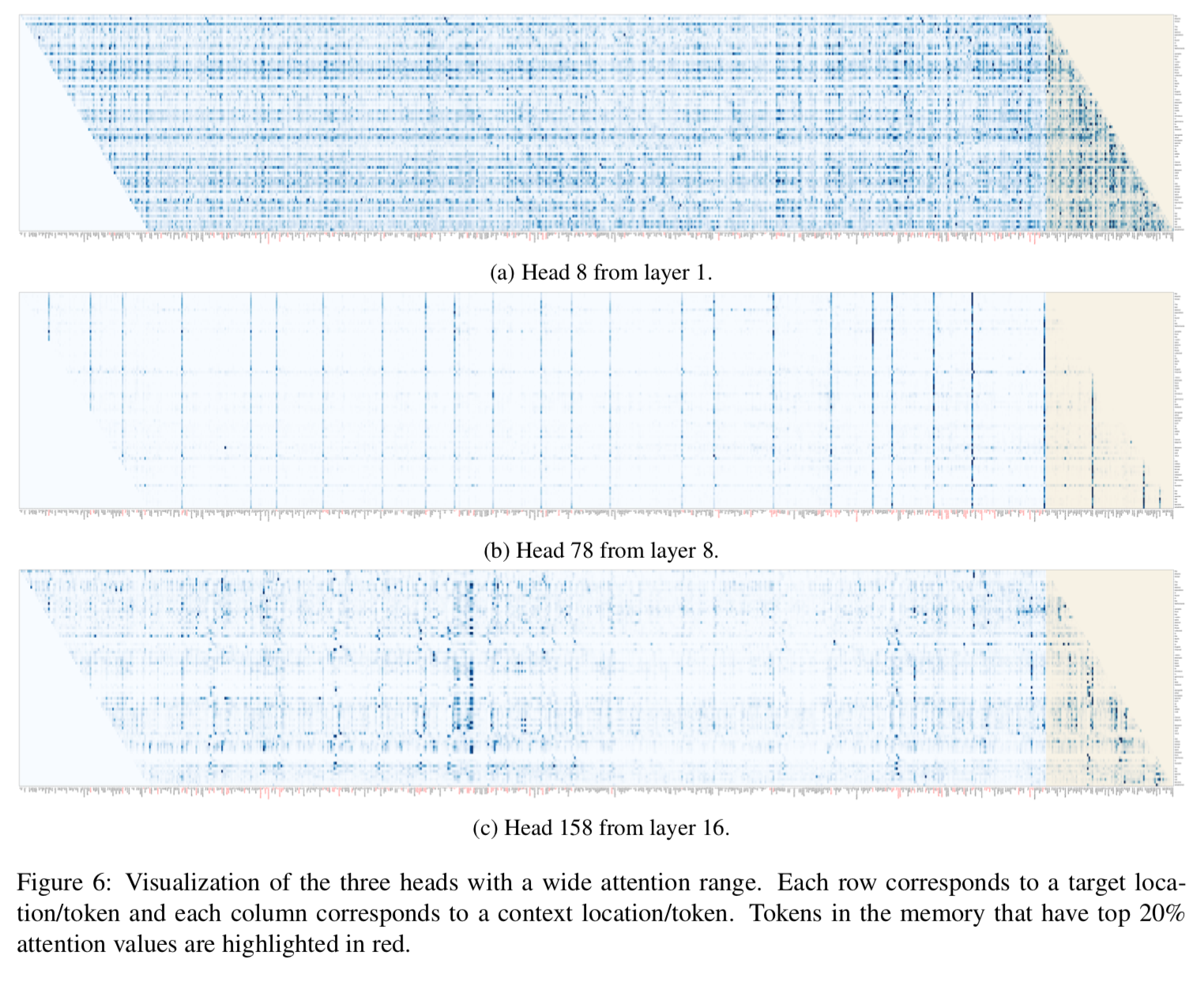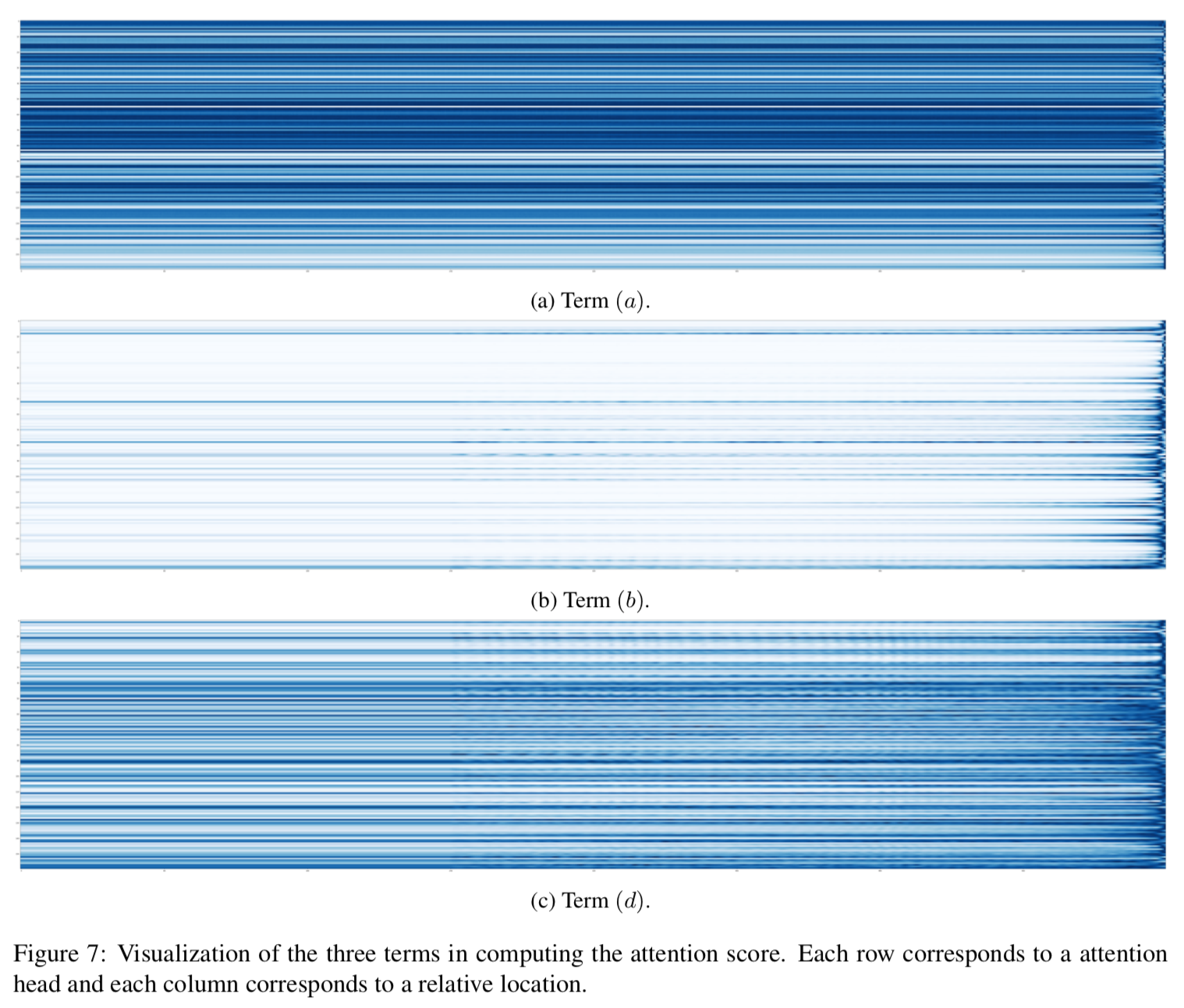$(c)$因为反映的是键本身的重要性，和context无关，这里不做考虑。从图中明显看到，$(b)$和之前的全局attention分布非常相似，揭示了全局attention很大程度上是由这一项决定的。当然，$(a)$中虽然很多地方是均匀分布，但是可以看到某些head是尖分布的，这应该是近义词间的attention。

### XLNet

#### 简介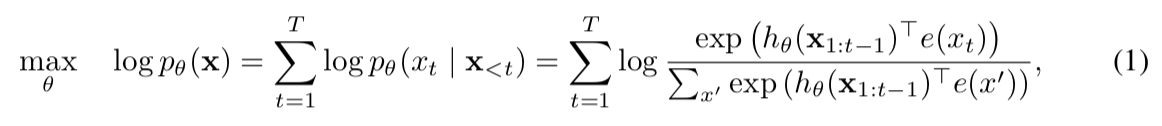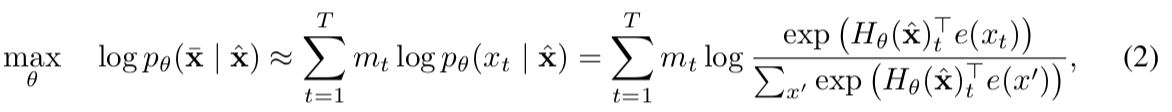AR和AE的目标函数比较，(2)中$\bar{x}$表示被masked的tokens，$\hat{x}$则是经过MASK处理后的带噪输入。注意(2)的$\approx$强调了这里面有独立假设导致等号不成立。

XLNet给出的方案是：通过打乱文本序列的token顺序，使得用前n-1的token来预测第n个token时，能同时用到上下文的信息，对所有可能的token排列（all possible permutations of the factorization order）来求期望的对数似然函数。这可以看作是基于排序的AR建模（permutation-based AR modeling），类似的做法在早前已经被人提出，不过出发点不同，之前的目标是希望通过无序模型来提升估计效果，建模时没有考虑原本的位置信息（orderless，退化成bag-of-words）。而XLNet的出发点是希望让AR能够学习到双向contexts，提高pretrain和fine-tune的匹配，位置信息是被考虑在内的。具体做法见下文。

#### 模型框架

##### Ojbective: Permutation Language Modeling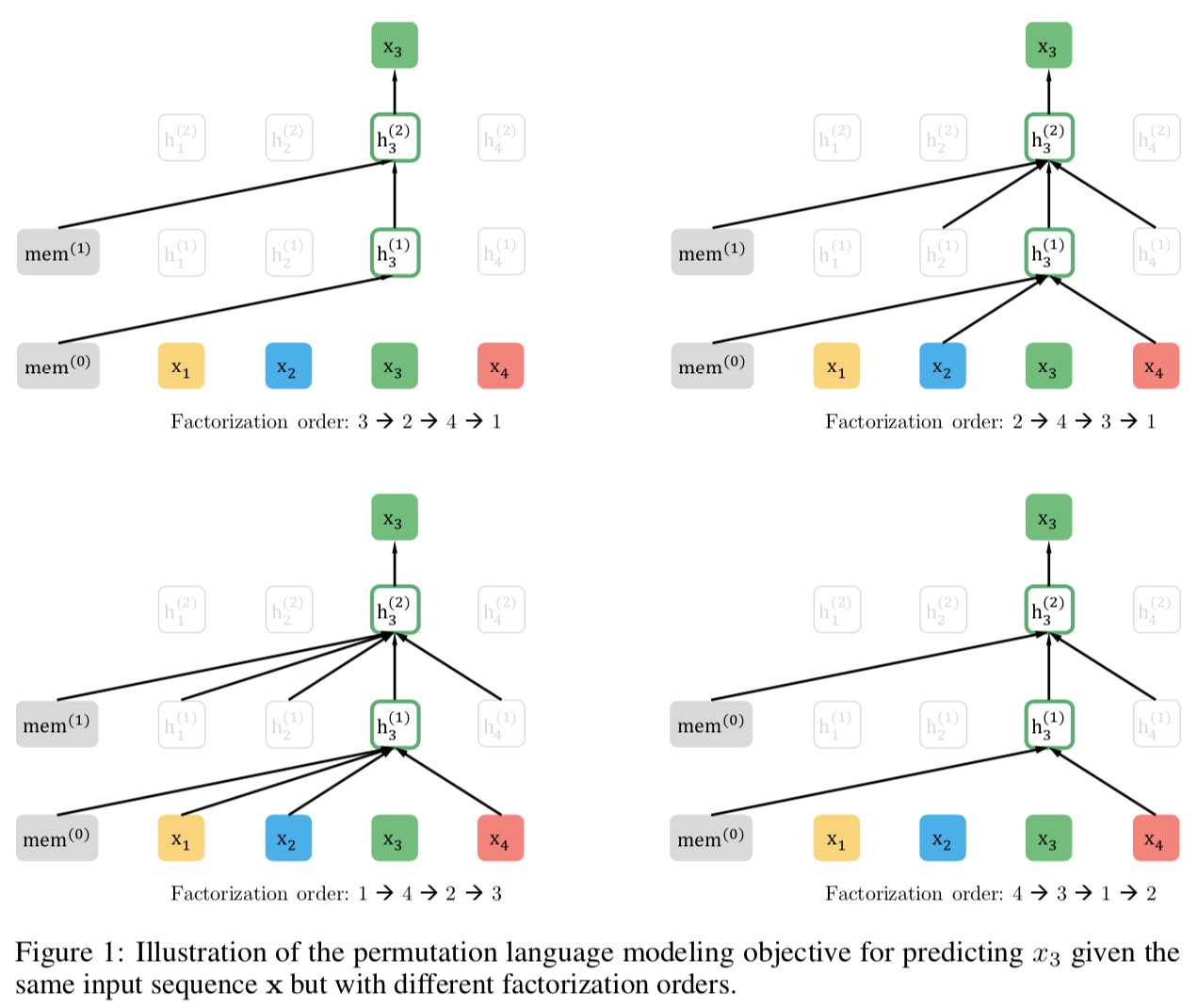PLM的目标函数可以表示如下：##### Architecture: Two-Stream Self-Attention for Target-Aware Representations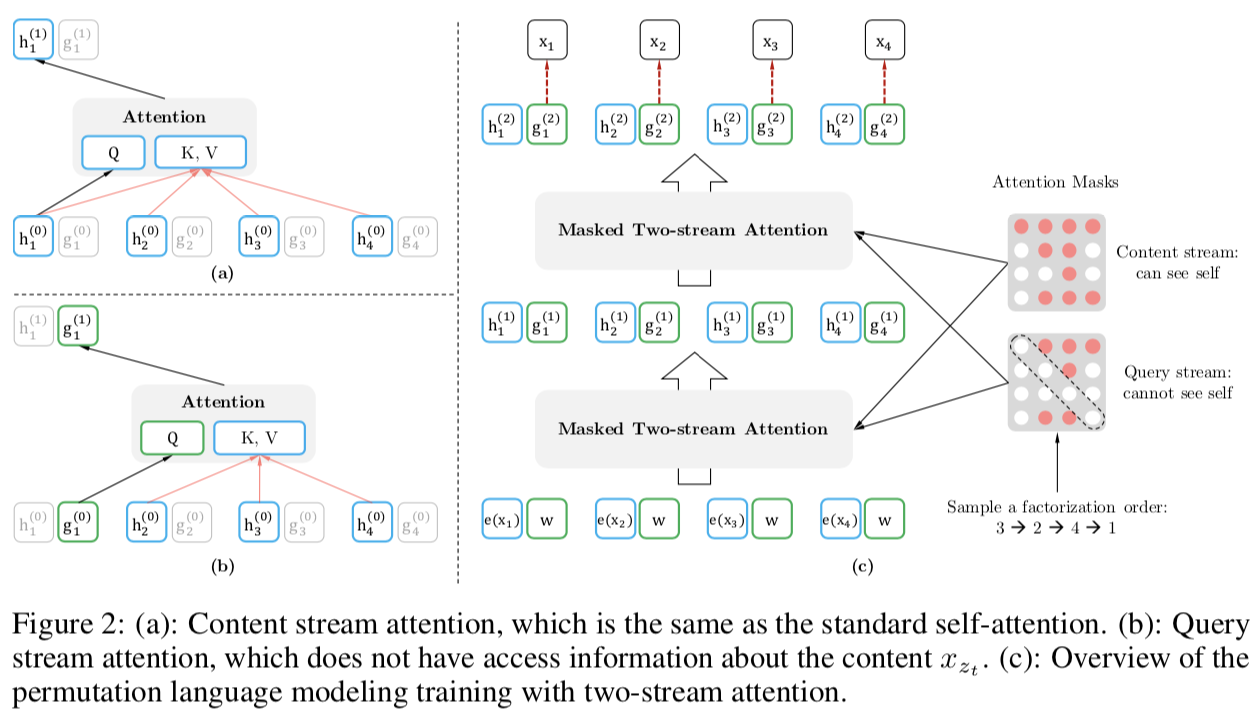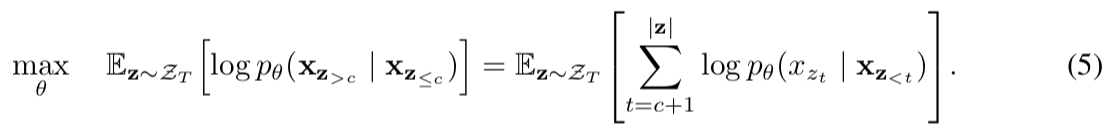##### Examples

BERT和XLNet看上去都只进行了部分预测（partial prediction），但是如之前所说，BERT无法建模targets之间的依赖（dependency）是硬伤。“打蛇打七寸”，在和BERT对比中，作者给了一个直击要害的例子。#### 实验分析